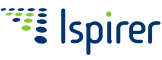Please, note, that Ispirer SQLWays 6.0 is no longer supported and provided to clients.
Check out the relevant toolkit documentation.
Ispirer Database Conversion overview.

# Ispirer SQLWays Database Migration Software

##### Returning Substring from String

This subsection describes functions returning a substring of a string, in various databases and their conversion by SQLWays..

TABLE 58. Returning a Substring from String
Database
Syntax
Description
Oracle
```SUBSTR (exp1, exp2
[,exp3])
```
Returns a substring of a string (exp1), beginning from exp2 with length of exp3.

� If exp2 is 0, then it is treated as 1.
� If exp2 is positive, then Oracle counts from the beginning of string to find the first character.
� If exp2 is negative, then Oracle counts backward from the end of string.
� If exp3 is omitted, then Oracle returns all characters to the end of string.
� If exp3 is less than 1, then a null is returned.

Return type: the same as exp1.
exp1 can be any of the datatypes CHAR, VARCHAR2, NCHAR, NVARCHAR2, CLOB, or NCLOB.

Floating-point numbers passed as arguments to SUBSTR are automatically converted to integers.
Returns a substring of a string (exp1), beginning from exp2 with length of exp3.
If any argument is null, the result is the null value.

Return type: the same as exp1.

exp2 must be an integer between 1 and the length or maximum length of exp1, depending on whether exp1 is fixed-length or varying-length.

exp3 is an expression that specifies the length of the result. If specified, exp3 must be a binary integer in the range 0 to n, where n equals (the length attribute of exp1) - exp2 + 1.
The default for exp3 is the number of bytes from the byte specified by the exp2 to the last byte of exp1 in the case of character string or binary string or the number of double-byte characters from the character specified by the exp2 to the last character of exp1 in the case of a graphic string. However, if exp1 is a varying-length string with a length less than exp2, the default is zero and the result is the empty string.
IBM DB2
```SUBSTR (exp1, exp2
[,exp3])
```
MySQL
```SUBSTRING (exp1,
exp2 [,exp3])
SUBSTRING (exp1
FROM exp2 [FOR
exp3])
```

Returns a substring of a string (exp1), beginning from exp2 with length of exp3.

Return type: the same as exp1.

� If exp2 is positive, then SUBSTRING function counts from the beginning of string to find the first character.
� If exp2 is negative, then SUBSTRING function counts backward from the end of string. (Undocumented).
� If exp3 is omitted, then SUBSTRING function returns a substring from string exp1 starting at position exp2.
Microsoft SQL Server

```SUBSTRING (exp1,
exp2, exp3)
```
Returns a substring of a string (exp1), beginning from exp2 with length of exp3.

exp1 - is a character string, binary string, text, image, a column, or an expression that includes a column.

exp2 - is an integer that specifies where the substring begins.

exp3 - is an integer that specifies the length of the substring (the number of characters or bytes to return).

Return type:
� If expression (exp1) is one of the character data types then function returns character data.
� If expression (exp1) is one of the binary data types then function returns binary data.
� The returned string is the same type as the given expression (exp1) with the exceptions shown in the following table:
given expression (exp1)
return type
text
varchar
image
varbinary
ntext
nvarchar
```RIGHT (char_exp,
int_exp)
```
Returns a substring of a string (char_exp). Returning substring contains int_exp last symbols.

Return type: varchar.

int_exp is an expression that specifies the length of the result.
```LEFT    (char_exp,
int_exp)
```
Returns a substring of a string (char_exp). Returning substring contains int_exp first symbols.
Return type: varchar.
int_exp is an expression that specifies the length of the result.